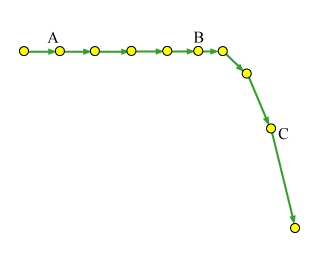# Problem: To understand the meaning and the basic applications of Newton's 1st and 2nd laws. In this problem, you are given a diagram (Figure 1) representing the motion of an object--a motion diagram. The dots represent the object's position at moments separated by equal intervals of time. The dots are connected by arrows representing the object's average velocity during the corresponding time interval. Your goal is to use this motion diagram to determine the direction of the net force acting on the object. You will then determine which force diagrams and which situations may correspond to such a motion. Which of these situations describe the motion shown in the motion diagram at point C?

###### FREE Expert Solution

Force, F = ma

At point A, a = 0.

89% (445 ratings)###### Problem Details

To understand the meaning and the basic applications of Newton's 1st and 2nd laws. In this problem, you are given a diagram (Figure 1) representing the motion of an object--a motion diagram. The dots represent the object's position at moments separated by equal intervals of time. The dots are connected by arrows representing the object's average velocity during the corresponding time interval. Your goal is to use this motion diagram to determine the direction of the net force acting on the object. You will then determine which force diagrams and which situations may correspond to such a motion. Which of these situations describe the motion shown in the motion diagram at point C?